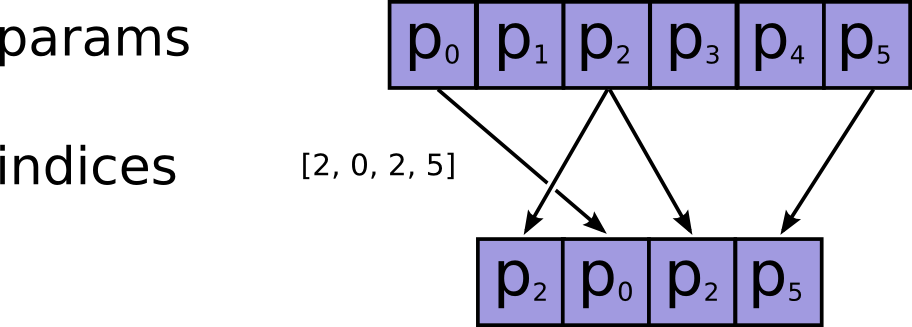# tf.compat.v1.gather

Gather slices from params axis axis according to indices.

Gather slices from params axis axis according to indices. indices must be an integer tensor of any dimension (usually 0-D or 1-D).

For 0-D (scalar) indices:

\begin{align*} output[p_0, ..., p_{axis-1}, && &&& p_{axis + 1}, ..., p_{N-1}] = \\ params[p_0, ..., p_{axis-1}, && indices, &&& p_{axis + 1}, ..., p_{N-1}] \end{align*}

Where N = ndims(params).

For 1-D (vector) indices with batch_dims=0:

\begin{align*} output[p_0, ..., p_{axis-1}, && &i, &&p_{axis + 1}, ..., p_{N-1}] =\\ params[p_0, ..., p_{axis-1}, && indices[&i], &&p_{axis + 1}, ..., p_{N-1}] \end{align*}

In the general case, produces an output tensor where:

\begin{align*} output[p_0, &..., p_{axis-1}, & &i_{B}, ..., i_{M-1}, & p_{axis + 1}, &..., p_{N-1}] = \\ params[p_0, &..., p_{axis-1}, & indices[p_0, ..., p_{B-1}, &i_{B}, ..., i_{M-1}], & p_{axis + 1}, &..., p_{N-1}] \end{align*}

Where N = ndims(params), M = ndims(indices), and B = batch_dims. Note that params.shape[:batch_dims] must be identical to indices.shape[:batch_dims].

The shape of the output tensor is:

output.shape = params.shape[:axis] + indices.shape[batch_dims:] + params.shape[axis + 1:].

Note that on CPU, if an out of bound index is found, an error is returned. On GPU, if an out of bound index is found, a 0 is stored in the corresponding output value.

See also tf.gather_nd.params The Tensor from which to gather values. Must be at least rank axis + 1.
indices The index Tensor. Must be one of the following types: int32, int64. Must be in range [0, params.shape[axis]).
validate_indices Deprecated, does nothing.
axis A Tensor. Must be one of the following types: int32, int64. The axis in params to gather indices from. Must be greater than or equal to batch_dims. Defaults to the first non-batch dimension. Supports negative indexes.
batch_dims An integer. The number of batch dimensions. Must be less than rank(indices).
name A name for the operation (optional).

A Tensor. Has the same type as params.

[]
[]